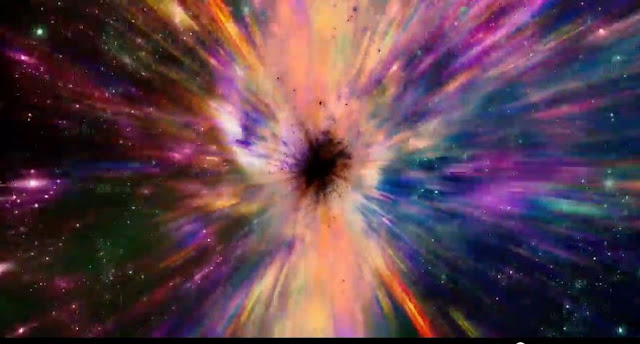## Thursday, November 16, 2017

### What Is the Computational Power of the Universe?

Can a close look at the universe give us solutions to problems too difficult even for a planet-sized computer to solve?Credit: NIST

In this video, The Computational Power of the Universe, National Institute of Standards and Technology physicist Stephen Jordan asks, “What if we consider the cosmos to be the output of a 13.7-billion-year computation?”

After all, computers crunch numbers to simulate complex change and the universe has undergone billions of years of change in accordance with the laws of nature. Jordan isn’t looking to convert the entire cosmos into a vast computing device (however marvelous a science-fiction premise that idea might make) but he is examining whether or not we can use what we see through our telescopes to gain insights into difficult computational problems.

Jordan applies this concept to a computer-stumping question called the number partitioning problem(link is external): If you had a pile of millions of very large numbers and wanted to divide them into two equal piles, how would you do it? The math is so difficult that it’s been considered as a practical basis for cryptography.

As it turns out, the universe has already processed a similar problem physically. Everywhere you look, empty space has a background energy density that is very close to zero. This near-zero value, which Einstein referred to as the Cosmological Constant, implies that the balance between energy from different fields related to fundamental universal forces somehow got sorted out well enough that we ended up with a fairly stable material universe. In essence, we live in a particular solution to partitioning.

Are there other tough problems out there to which the universe holds a shortcut? To be continued.

Contacts and sources: